Share
Any question? Call us at : 09 70 44 94 99
THE ART OF AFRICAN CRAFTS QUALITY
0
item
0.00 € VAT Incl.
Your shopping cart
Products search Advanced search

# To know the ring size ?

Important
We set up our own international system of classification of the sizes conscript '' Nanadiany sizes '' (N). This particular system includes all the sizes of rings of the whole world. Look below to the system of your country to find the equivalent of your right size at Nanadiany
French and European size
By means of this board chose the equivalent of your French or European Size at Nanadiany.

Nanadiany size = French or European Size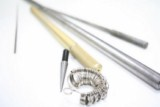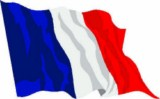N7 = 42 N11 = 44 N13 = 45 N16 = 46 N18 = 47 N19 = 48 N20 = 49 N22 = 50 N25 = 51 N27 = 52 N30 = 53 N33 = 54 N35 = 55 N37 = 56 N39 = 57 N41 = 58 N43 = 59 N45 = 60 N47 = 61 N49 = 62 N51 = 63 N53 = 64 N55 = 65 N58 = 66
USA and Canada size
By means of this board chose the equivalent of your USA and Canada Size at Nanadiany.

Nanadiany size = USA and Canada Size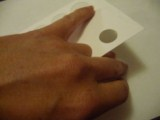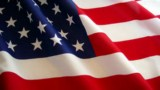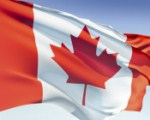N1 = 0.5 (1/2) N3 = 1 N5 = 1 1/2 N7 = 2 N9 = 2 1/2 N11 = 3 N14 = 3 1/2 N17 = 4 N19 = 4 1/2 N21 = 5 N24 = 5 1/2 N26 = 6 N30 = 6 1/2 N34 = 7 N37 = 7 1/2 N39 = 8 N42 = 8 1/2 N44 = 9 N47 = 9 1/2 N49 = 10 N52 = 10 1/2 N54 = 11 N57 = 11 1/2
English ( UK) size
By means of this board chose the equivalent of your English (UK) Size at Nanadiany.

Nanadiany size = English (UK) Size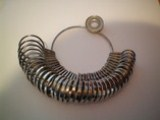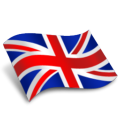N1 = A N3 = B N5 = C N7 = D N9 = E N11 = F N14 = G N16 = H N18 = I N20 = J N22 = K N24 = L N26 = M N30 = N N34 = O N37 = P N39 = Q N45 = S N48 = T N50 = U N53 = V N55 = W N58 = X
German size
By means of this board chose the equivalent of your German Size at Nanadiany.

Nanadiany size = German Size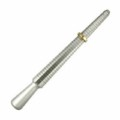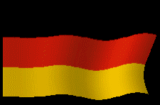N7 = 13 1/2 N11 = 14 N17 = 15 N20 = 15 1/2 N23 = 16 N26 = 16 1/2 N30 = 17 N34 = 17 1/4 N37 = 17 3/4 N39 = 18 N42 =18 1/2 N44 = 19 N47 = 19 1/2 N49 = 20 N57 = 21
Nanadiany size with the internal ring diameter in mm
Size of rings
Checking the sizes : if you have got already a ring that suits you, measure the internal diameter and consult the chart. You will see the number corresponding to the size of your ring. You must give the chosen number while making an order.
Notice: if the received ring is too big, try to put one or several coatings of nail-polish (incolor or other) inside the ring in order to thicken a little. Or you can change just the finger ... ;-)

Nanadiany size = ring internal diameter in mm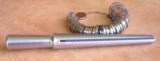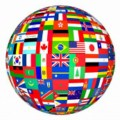N1 = 11.0 N2 = 12.1 N3 = 12.3 N4 = 12.6 N5 = 12.7 N6 = 13.0 N7 = 13.2 N8 = 13.4 N9 = 13.6 N10 = 13.8 N11 = 14.0 N12 = 14.1 N13 = 14.3 N15 = 14.5 N16 = 14.6 N17 = 14.8 N18 = 15.0 N19 = 15.2 N20 = 15.4 N21 = 15.7 N22 = 15.8 N23 = 16.0 N24 = 16.1 N25 = 16.4 N26 = 16.5 N28 = 16.6 N29 = 16.7 N30 = 16.8 N31 = 17.0 N32 = 17.1 N33 = 17.2 N34 = 17.3 N35 = 17.4 N36 = 17.6 N37 = 17.7 N38 = 18.0 N39 = 18.1 N40 = 18.3 N41 = 18.5 N42 = 18.6 N43 = 18.8 N44 = 19.0 N45 = 19.1 N46 = 19.3 N47 = 19.5 N48 = 19.6 N49 = 19.8 N50 = 20.0 N51 = 20.0 N52 = 20.2 N53 = 20.3 N54= 20.7 N55 = 20.8 N56 = 20.9 N57 = 21.1 N58 = 21.2